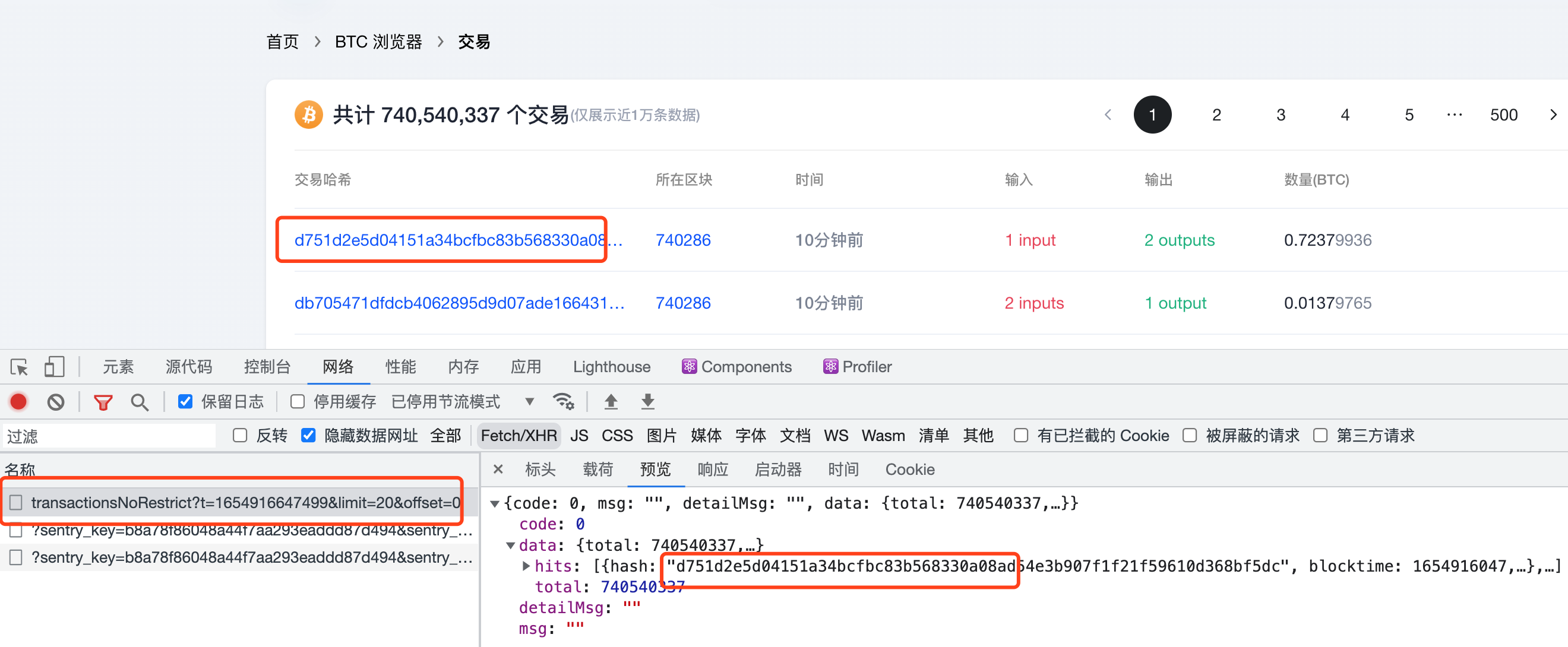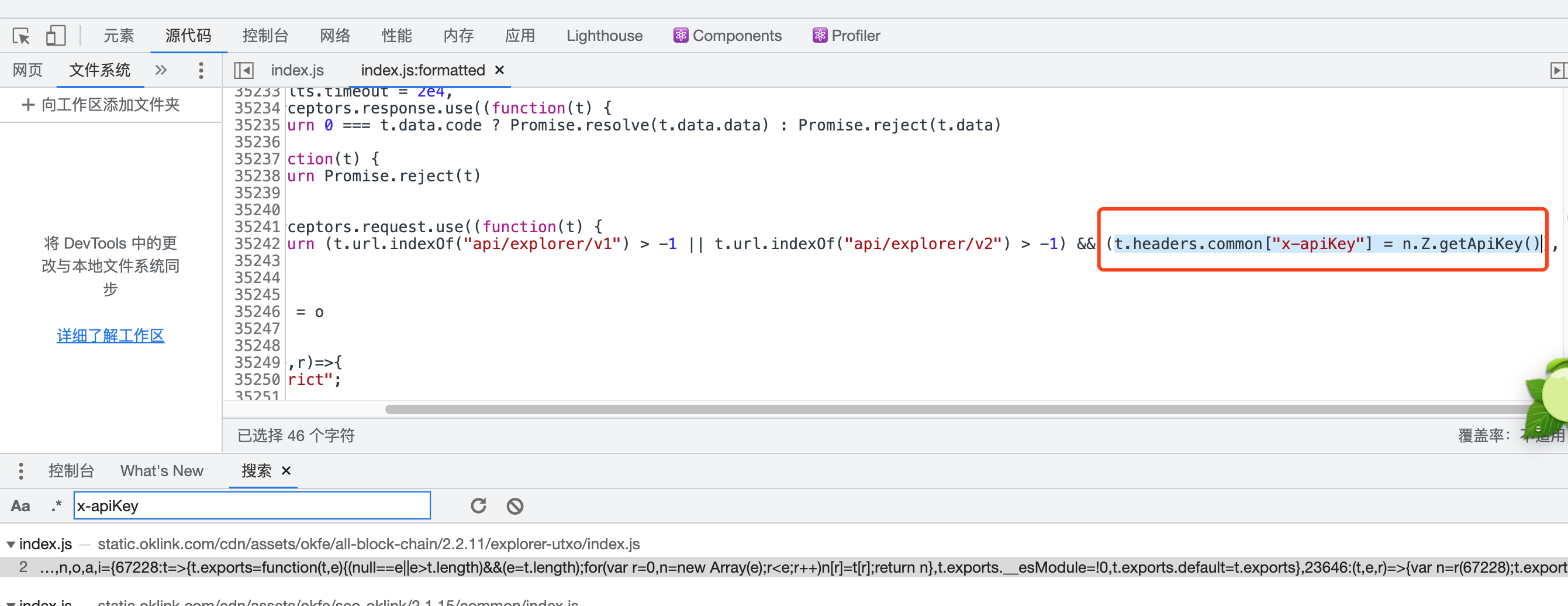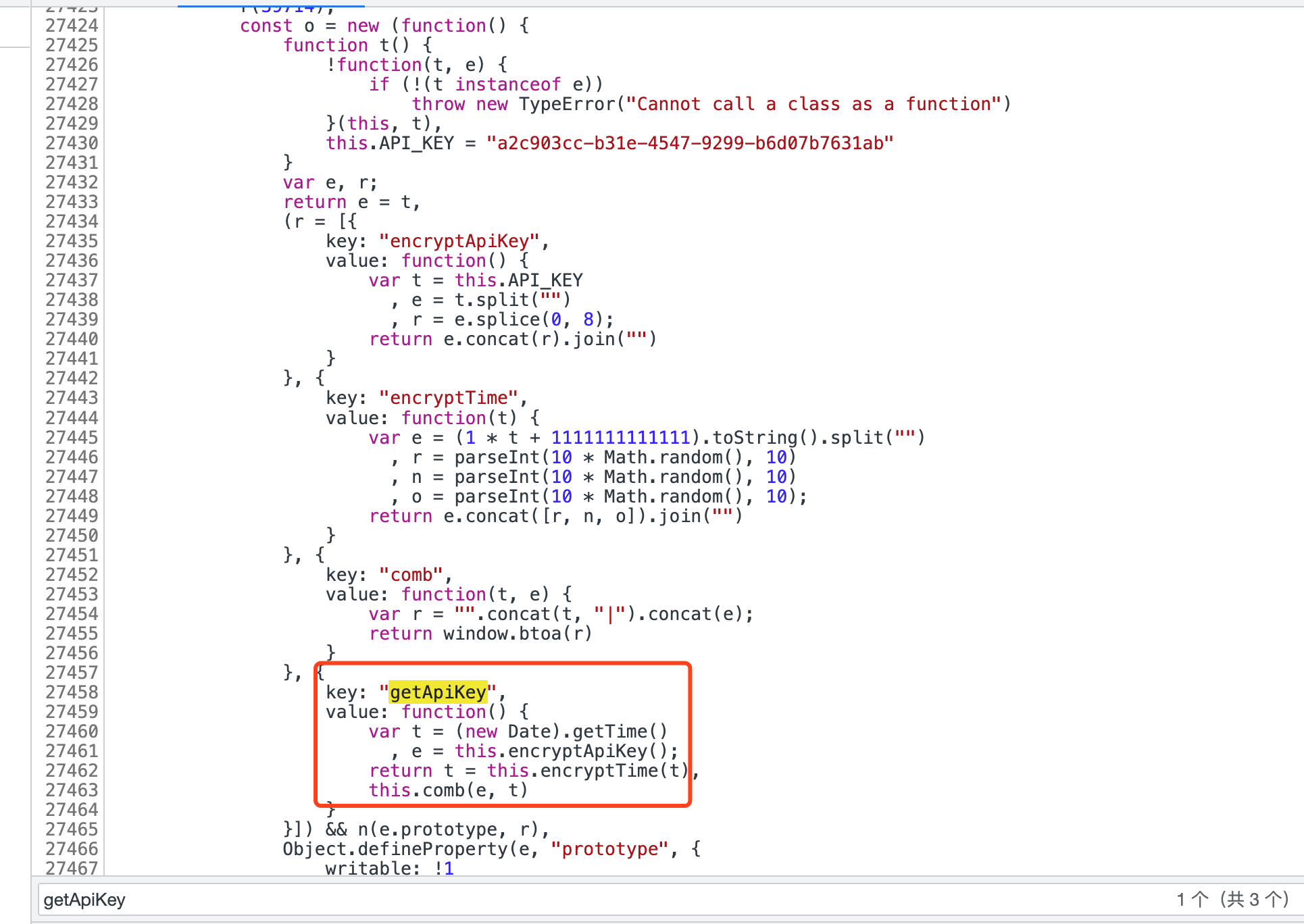python爬虫之JS逆向
tjk
2022-06-22 17:42:18 2022-06-22
17
0

Python爬虫之JS逆向案例

JS逆向有两种思路：``t.headers.common["x-apiKey"] = n.Z.getApiKey()``1.接下就是解析这个getApiKey方法了。

``{                    key: "getApiKey",                    value: function() {                        var t = (new Date).getTime()                          , e = this.encryptApiKey();                        return t = this.encryptTime(t),                        this.comb(e, t)                    }                }``

1).变量t就是获取当前时间戳

2).变量e是调用encryptApiKey这个方法

3).变量t是encryptTime(t)这个方法处理后的返回

4).最后通过comb(e,t)个方法生成最终的apiKey

2.encryptTime方法

``key: "encryptApiKey",                    value: function() {                        var t = this.API_KEY                          , e = t.split("")                          , r = e.splice(0, 8);                        return e.concat(r).join("")                    }``

1).变量t是一个常量字符串API_KEY,往上找到初始化值为this.API_KEY = "a2c903cc-b31e-4547-9299-b6d07b7631ab"

2).变量e是将上面的t分隔成数组

3).变量r：从变量e中删除前8个字符串，并将e的前8个元素组成的数组赋值给r。同时变量e删除了前8个元素

4).最终将e和r合并在一起并转为字符串

3.encryptTime方法

``key: "encryptTime",                    value: function(t) {                        var e = (1 * t + 1111111111111).toString().split("")                          , r = parseInt(10 * Math.random(), 10)                          , n = parseInt(10 * Math.random(), 10)                          , o = parseInt(10 * Math.random(), 10);                        return e.concat([r, n, o]).join("")                    }``

1).变量e为将入参t加上1111111111111然后转为字符串，分隔为数组

2).变量r、n、o三个是生成10以内的随机整数

3).最后返回的是e和[r,n,o]数组合并，转为字符串返回

4.comb方法

``key: "comb",                    value: function(t, e) {                        var r = "".concat(t, "|").concat(e);                        return window.btoa(r)                    }``

1).变量r是由入参t和e中间加上|然后拼在一起的

2).window.btoa是返回的base64加密编码

3)comb方法最终返回的就是我们需要的x-apiKey的值了

5.将上面的JS逻辑转为Python代码如下：

``def get_api_key():    cur_time = int(time.time() * 1000)    api_key = 'a2c903cc-b31e-4547-9299-b6d07b7631ab'    key_1 = api_key[0:8]    key_2 = api_key[8:]    encrypt_api_key = key_2 + key_1    string = str(cur_time + 1111111111111)    r = random.randint(0, 9)    n = random.randint(0, 9)    o = random.randint(0, 9)    encrypt_time = '%s%s%s%s' % (string, r, n, o)    new_key = encrypt_api_key + '|' + encrypt_time    # 转为bytes-like object    new_key = new_key.encode('utf-8')    # 将bytes-like object转成字符串类型    return str(base64.b64encode(new_key), encoding='utf-8')``